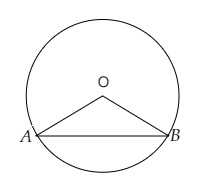# In a circle of radius $6 \mathrm{~cm}$, a chord of length $10 \mathrm{~cm}$ makes an angle of $110^{\circ}$ at the centre of the circle. Find the circumference of the circle.

Given:

Radius of the circle $r=6 \mathrm{~cm}$.

Length of the arc $l=10 \mathrm{~cm}$.

Angle subtended at the centre $=110^{\circ}$.

To do:

We have to find the circumference of the circle.

Solution:Let $OA$ and $OB$ are the radii of the circle and $AB$ the chord.

We know that,

Circumference of a circle of radius $r$ is $2 \pi r$.

Therefore,

Circumference of the circle $=2 \times 3.14 \times 6\ cm$

$=37.68\ cm$

The circumference of the circle is $37.68\ cm$.

Updated on: 10-Oct-2022

27 Views